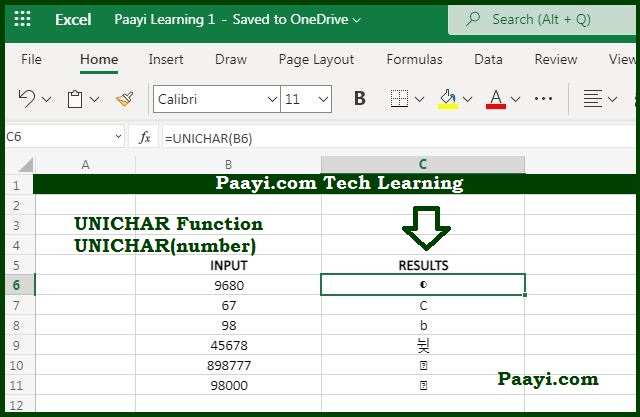# Learn How to Use Microsoft Excel UNICHAR Function - Done

Written by | 0 Comments | 677 Views

In this article, you will learn how to use the MS Excel UNICHAR function and its prime function in Microsoft Excel. You will also get to know the Microsoft Excel UNICHAR function return value and syntax with the help of some examples.

## Microsoft Excel UNICHAR Function

The primary function of the Microsoft Excel UNICHAR character is to get a Unicode character by a number. That means with the help of the UNICHAR function; you can able to return a Unicode character based on a given number.

### UNICHAR Function Return Value

It will return the Unicode character.

### UNICHAR Function Syntax

= UNICHAR(number)

Where the argument:

• number: It is the code point for a Unicode character in decimal.

## How to Use the Microsoft Excel UNICHAR Function?As we know, the UNICHAR function is used to return a Unicode character from a given code point, provided as a decimal value. Unicode is the computer standard used for the unified coding, text-handling, and representation in most of the world's writing systems.

Let us understand the UNICHAR function with the help of the example given below:

Example 1: The Euro Symbol has code point 8364 in the decimal notation, the formula used below will return the Euro character:

= UNICHAR(8364) - It will return the Euro sign.